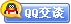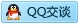##用户名 Email 自动登录 找回密码 密码 会员注册
 VIP会员，3年作业免费下 ！ 奥鹏作业，奥鹏毕业论文检测 新手作业下载教程，充值问题 没有找到答案，请在此处留言！ 2020年07月最新全国统考资料 投诉建议，加盟合作！奥鹏课程积分软件(ver:3.1)

# 东北大学12秋学期《JAVA语言程序设计Ⅰ》在线作业2发表于 2012-12-18 15:08:18 | 显示全部楼层 |阅读模式谋学网: www.mouxue.com 主要提供奥鹏作业答案，奥鹏12秋在线作业答案，奥鹏离线作业答案和奥鹏毕业论文以及提供代做作业服务，致力打造中国最专业远程教育辅导社区) M* ]+ k0 _( `( E1 C6 F + h: t9 |( C1 g2 i5 L7 a( o" Z 一、单选题（共 20 道试题，共 60 分。）V 1.  已知如下代码： public class Test { long a[] = new long; public static void main ( String arg[] ) { System.out.println ( a ); } } 请问哪个语句是正确的？; a( ~\$ D6 k3 h+ y) R\$ U) t3 b% _ A. 输出结果是 null. B. 输出结果是0 C. 编译时出错0 ~) a  p+ U- e! u+ p  @" ~ D. 运行时出错* w2 n' J  d. Q+ b4 Q       满分：3  分 2.  若a的值为3时，下列程序段被执行后，c的值是多少？（ ） c = 1; if ( a>0 ) if ( a>3 ) c = 2; else c = 3; else c = 4; A. 13 \* {" R* U5 v' e B. 2' L, n6 ?7 s3 u3 m C. 3+ R4 b3 @( C6 y5 ^3 n1 p: Y D. 4       满分：3  分 3.  下列代码中，将引起一个编译错误的行是 1）public class Test{ 2） int m,n; 3） public Test() {} 4） public Test(int a) {m=a;} 5） public static void main(String args[]){ 6） Test t1,t2; 7） int j,k; 8） j=0;k=0; 9） t1=new Test(); 10） t2=new Test(j,k); 11） } 12  C% ^# O/ H* x4 C\$ T6 R" q2 L* P A. 第3行" d: a+ ^( h" z2 @1 I. H6 S* z. M B. 第5行/ R% P) B  {8 Z9 {, h C. 第6行, o, }7 e. O) A\$ b6 O  G6 ^ D. 第10行2 G3 m  U4 E2 D5 u/ p/ J       满分：3  分+ ?7 B  C' ^. m2 _* p5 Y9 Y 4.  已知如下代码： boolean m = true; if ( m = false ) System.out.println("False"); else System.out.println("True"); 执行结果是什么？( Q6 O# v# O6 N# `& | A. False B. True3 z8 {* a% S; d: }* Q5 n; d C. 编译时出错 D. 运行时出错       满分：3  分 5.  下面的语句的作用是：（ ）。 Vector MyVector = new Vector(100,50);1 s+ w9 c" H& O9 a+ [- J/ r A. 创建一个数组类对象MyVector，有100个元素的空间，每个元素的初值为50。" Q+ Q" u, d* P; H B. 创建一个向量类对象MyVector，有100个元素的空间，每个元素的初值为50。* z/ c0 D& g/ h  d2 J1 q) Z C. 创建一个数组类对象MyVector，有100个元素的空间，若空间使用完时，以50个元素空间单位递增。 D. 创建一个向量类对象MyVector，有100个元素的空间，若空间使用完时，以50个元素空间单位递增。% y1 S. V) v0 v+ V& l7 c       满分：3  分+ `2 m1 z8 p& ~, @' @# B 6.  在Java中，由Java编译器自动导入，而无需在程序中用import导入的包是8 A8 }3 N! T* f\$ O/ }, M A. java.applet B. java.awt C. java.util+ f/ v# j; v# \\$ Q+ k) N D. java.lang- m; g) }5 s\$ p       满分：3  分8 s\$ \( q* a9 }, s) m/ U. w 7.  使用那个关键字，使得只有在定义该类的包中的其他类才能访问该类？. j7 [6 S" \5 E& i: ]& [5 L. y A. 不使用关键字& \7 M, l& v6 B7 Q) z% Z B. private C. final1 Z& C: n9 e! y3 C0 G9 u; X D. protected       满分：3  分2 j5 M8 U4 O' a. o 8.  使用哪一个修饰符才能阻止一个类被继承？. p' U, t" m! b A. final B. protected- H" i3 o5 R* P C. private; e) p! E4 |) @1 I' x7 ]9 n2 E D. abstract       满分：3  分 9.  下列语句序列执行后，k 的值是( )。 int x=6, y=10, k=5; switch( x%y ) { case 0: k=x*y; case 6: k=x/y; case 12: k=x-y; default: k=x*y-x; }- `7 I0 d" O% U: d7 C1 n( u A. 60. w) q6 d8 V2 E. y3 ^- ]5 z0 J9 O B. 547 m( ~5 _* b3 S C. 09 k- \! X& k0 x D. 5" q" G% @8 I. Y  q3 k* g  V       满分：3  分 10.  在程序的源文件开始处有下面一行程序： package awt;' [" C+ x6 G) J A. 结果是一个编译错误，因为Java已经定义了一个awt包  [8 ]2 u9 O4 l& H1 `" T. V. s B. 说明这个文件里的所有的类都应该包含在java.awt包里( z1 K4 U5 ~* i! x0 z' H C. 说明这个文件里的所有的类都应该包含在自己定义的awt包里2 W( r\$ k, S# b% b8 o% Y% x D. 导入你自己定义的awt包里的所有类       满分：3  分 11.  Java的字符类型采用的是Unicode编码方案，每个Unicode码占用（ ）个比特位。& F, S; p1 I! L# t# l9 Z A. 8 B. 162 H9 X+ R# _, ?) e' x C. 32' g! f) T" U" ^ D. 649 s( _9 e* d7 x1 |7 I; S# p* H       满分：3  分' J4 L0 d* j1 |6 j4 G. d 12.  表达式5&2的值应该是： A. 04 m% e% A/ z! R" v7 b\$ N B. 26 `8 c5 c6 C1 D. v# Z) w8 q7 o% E C. 56 H0 `0 g8 [: c9 g D. 7. H, i7 g+ M( n/ L       满分：3  分 13.  如果类中的成员变量可以被同一包访问，则使用如下哪个约束符?  s/ I\$ K3 |2 w/ u A. private B. public C. protected D. 不使用约束符9 ?) ^0 P( E, n- Z0 Q3 V) V       满分：3  分; `9 M! y3 i  L5 I 14.  下列类头定义中，错误的是( )。( i- q" J3 b( w0 \ A. class x { .... }+ |# ]5 r( y  h% F B. public x extends y { .... }# J4 J% m% K- r4 d- ^; F C. public class x extends y { .... }' M6 `\$ [- B\$ k" q/ X! j D. class x extends y implements y1 { .... }& E  Y' @6 u. a       满分：3  分 15.  如果A类基于B类，请问哪个类是子类，哪个类是父类？, p* x: \! K3 P+ G, l A. 两个都不是父类或者子类 B. A是B的子类" e\$ J  Y\$ I0 D% K2 \\$ x7 L9 I C. B是A的子类 D. 两个都是对方的子类- E9 C0 B8 @\$ ^3 \       满分：3  分( f7 l& C+ a+ b) u8 | 16.  下面哪一个能够正确的隐藏方法 void hai(int a,int b)？ A. public void hai(int a,int b) B. public hai(int a)7 P9 B+ w. h2 I C. int hai(int c, int d) D. int hai(String s)       满分：3  分; W* [0 @% ^0 V+ J 17.  下列关于内部类的说法不正确的是 A. 内部类的类名只能在定义它的类或程序段中或在表达式内部匿名使用 B. 内部类可以使用它所在类的静态成员变量和实例成员变量 C. 内部类不可以用abstract修饰符定义为抽象类; o+ P2 m3 N& D D. 内部类可作为其他类的成员，而且可访问它所在类的成员  q7 T( o2 x3 }2 x1 V: Q- p       满分：3  分 18.  以下代码的输出结果是什么? class Foo{ public static void main(String args[]){ int x=4,j=0; switch(x){ case 1:j++; case 2:j++; case 3:j++; case 4:j++; case 5:j++; break; default:j++; } System.out.println(j); } } A. 1: C; z" l% {* F; |3 j4 k7 J\$ p B. 2- D. B% p. B) N\$ D" v C. 3 D. 编译错误       满分：3  分 19.  已知如下代码： 1) class Example{ 2) String str; 3) public Example(){ 4) str= "example"; 5) } 6) public Example(String s){ 7) str=s; 8) } 9) } 10) class Demo extends Example{ 11) } 12) public class Test{ 13) public void f () { 14) Example ex = new Example("Good"' F# a& ?0 b" D" p; Q- k\$ d A. 第3行\$ e% H/ y* d, m4 Y2 T! I* ^ B. 第6行 C. 第10行 D. 第14行 E. 第15行: X6 a: T0 D) A9 a* n9 d& K* P       满分：3  分 20.  在编写异常处理的Java程序中，每个catch语句块都应该与( )语句块对应，使得用该语句块来启动Java的异常处理机制。 A. if - else1 f. ~0 T- Y9 B. `2 E% o B. switch C. try3 a1 @' T- |8 x D. throw3 I0 R" S" ^: Z! {3 P       满分：3  分 ) O% A2 z8 n+ G8 w 二、多选题（共 10 道试题，共 40 分。）V 1.  已知如下代码： public class Test { public static void main(String arg[]) { int i = 5; do { System.out.println(i); } while (--i>5) System.out.println("finished"); } } 执行后的输出结果包括什么？ A. 5 B. 45 Z+ y' w' P0 H) e\$ P- S2 b/ _ C. 6& o1 G* T& P1 z8 Y) C0 C D. finished E. 什么都不输出\$ g2 p; S2 }: z       满分：4  分8 G  h+ h2 w9 s  {+ h 2.  下面代码执行后的输出是什么？ outer: for(int i=0;i<3; i++) inner: for(int j=0;j<2;j++) { if(j==1) continue outer; System.out.println(j+ “ and “+i); } A. 0 and 0 B. 0 and 1- T# ~4 ?% ]7 o7 Z' p. B2 Z8 T C. 0 and 2/ h9 N\$ G- e9 J; g' k# S' C: Q: O( u D. 1 and 0 E. 1 and 12 k) f- \6 r6 o. x/ S0 } F. 1 and 2\$ a( K2 N* ?0 M) S+ n G. 2 and 0 H. 2 and 1 I. 2 and 2: V, `0 ~3 I6 P9 c- M. z       满分：4  分 3.  下面的哪些程序片断可能导致错误。8 |& r, X, ]  G A. String s="Gonewiththewind"; String t="good"; String k=s+t; B. String s="Gonewiththewind"; String t; t=s+"one";3 u5 i- O- `. l% [ C. String s="Gonewiththewind"; String standard=s.toUpperCase(); D. String s="homedirectory"; String t=s-"directory".       满分：4  分 4.  已知如下类定义： class Base { public Base (){ //... } public Base ( int m ){ //... } protected void fun( int n ){ //... } } public class Child extends Base{ // member methods } 如下哪句可以正确地加入子类中？ A. private void fun( int n ){ //...}& D5 F- R* Z5 ]! v9 H! P B. void fun ( int n ){ //... }% h3 f/ a* v/ C5 l- z  o; U, R! u C. protected void fun ( int n ) { //... }0 P7 a: w% s5 g D. public void fun ( int n ) { //... }       满分：4  分( O2 j- y: e/ M9 ]! T 5.  下面哪些不是java的原始数据类型。 A. short B. Boolean C. unit. P' j' K2 u  I8 z3 q* O- A3 G* b D. float& i/ W5 V2 B( X       满分：4  分, P3 r! n, u5 c( B 6.  以下哪个有关事件监听器的语句是正确的？3 y  d0 i" W; F. z0 d A. 多个监听器可以连接到一个组件上 B. 只有一个监听器可以连接到一个组件上* k: @+ ]/ d. ~( p; r& q7 c C. 一个监听器可以连接和控制多个组件 D. 一个监听器仅可以连接和控制一个组件: d! F: M& c& Y5 p; t       满分：4  分+ ]" W8 Q" p; i- i* N- W% v 7.  下面哪些不是Java中的容器?3 N& c9 a5 {  z, L6 d A. ScrollPane; @- R# Q5 l' D! H B. Canvas C. Scrollbar D. Applet' Y6 p! y8 i6 { E. Dialog       满分：4  分1 |* @# ]# S# G. X 8.  已知如下类说明： public class Test { private float f = 1.0f; int m = 12; static int n=1; public static void main(String arg[]) { Test t = new Test(); // 程序代码… } } 如下哪个使用是正确的？- o5 v\$ [, S- y8 l A. t.f3 L9 _" B2 z( t! M9 V\$ _! S B. this.n C. Test.m" U# p8 c# L0 [0 H% s. \ D. Test.n       满分：4  分' k# ]# {1 A6 x' I0 W9 F 9.  下面哪条语句能正确地创建一个2维整型数组？ A. int a[][] = new int[10,10]# i# D3 @4 J9 z- n! a- E6 O: [  u* G B. int a = new int[][];* U' p7 I- o3 ?% O& N3 w C. int a[][] = new int ; D. int []a[] = new int ;; w8 Z& i7 y. C       满分：4  分/ h. Y9 g& S1 ^" ^ 10.  已知如下类说明： public class Test { private float f = 1.0f; int m = 12; static int n=1; public static void main(String arg[]) { Test t = new Test(); // 程序代码… } } 如下哪个使用是正确的？ A. t.f, t% v& G; D& Y1 _- D9 w B. this.n C. Test.m+ u. o: L: p* S7 D/ k# U3 t  a D. Test.n. V\$ z# @  _5 n1 N0 \- C: B       满分：4  分 7 z  j( T+ N- u( Q' Q! f

### 本帖子中包含更多资源

x
 本版积分规则 回帖并转播 回帖后跳转到最后一页客服一客服二客服三客服四微信客服扫一扫